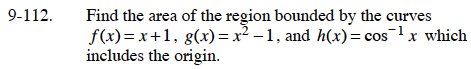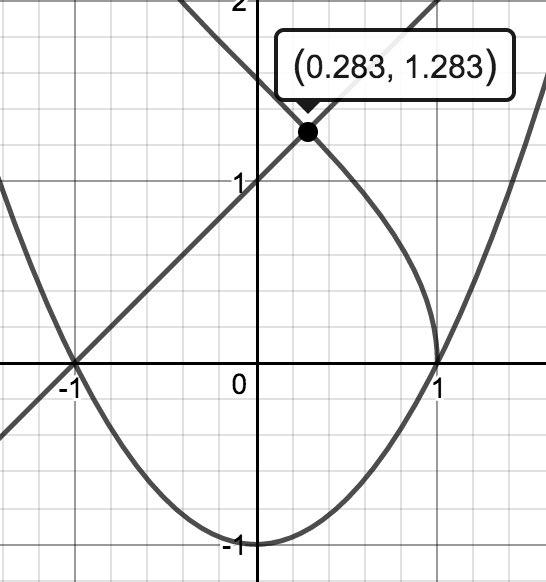### Home > CALC > Chapter 9 > Lesson 9.4.1 > Problem9-112

9-112.

Find the area of the region bounded by the curves f(x) = x + 1, g(x) = x2 − 1, and h(x) = cos−1 x which includes the origin. Homework Help ✎Graph the curves and determine the points of intersection.For the second integral, use integration by parts.
Let f = cos–1(x) and dg = 1dx.